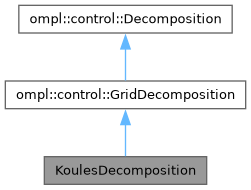KoulesDecomposition Class Reference
Inheritance diagram for KoulesDecomposition:[legend]

## Public Member Functions

KoulesDecomposition (const ompl::base::StateSpacePtr &space)

void project (const ompl::base::State *s, std::vector< double > &coord) const override
Project a given State to a set of coordinates in R^k, where k is the dimension of this Decomposition.

void sampleFullState (const ompl::base::StateSamplerPtr &sampler, const std::vector< double > &coord, ompl::base::State *s) const overridePublic Member Functions inherited from ompl::control::GridDecomposition
GridDecomposition (int len, int dim, const base::RealVectorBounds &b)
Constructor. Creates a GridDecomposition as a hypercube with a given dimension, side length, and bounds. The cells of the hypercube are referenced by integer coordinates of the form $$(r_1,\ldots,r_k)$$, where $$0 \leq r_i < \texttt{len}$$.

int getNumRegions () const override
Returns the number of regions in this Decomposition.

double getRegionVolume (int) override
Returns the volume of a given region in this Decomposition.

void getNeighbors (int rid, std::vector< int > &neighbors) const override
Stores a given region's neighbors into a given vector.

int locateRegion (const base::State *s) const override
Returns the index of the region containing a given State. Most often, this is obtained by first calling project(). Returns -1 if no region contains the State.

void sampleFromRegion (int rid, RNG &rng, std::vector< double > &coord) const override
Samples a projected coordinate from a given region.Public Member Functions inherited from ompl::control::Decomposition
Decomposition (int dim, const base::RealVectorBounds &b)
Constructor. Creates a Decomposition with a given dimension and a given set of bounds. Accepts as an optional argument a given number of regions.

virtual int getDimension () const
Returns the dimension of this Decomposition.

virtual const base::RealVectorBoundsgetBounds () const
Returns the bounds of this Decomposition.

virtual void sampleFullState (const base::StateSamplerPtr &sampler, const std::vector< double > &coord, base::State *s) const =0
Samples a State using a projected coordinate and a StateSampler.

## Static Public Member Functions

static ompl::base::RealVectorBounds bounds3 (const ompl::base::RealVectorBounds &bounds)Protected Member Functions inherited from ompl::control::GridDecomposition
virtual const base::RealVectorBoundsgetRegionBounds (int rid) const
Helper method to return the bounds of a given region.

void regionToGridCoord (int rid, std::vector< int > &coord) const
Converts a given region to a coordinate in the grid.

int gridCoordToRegion (const std::vector< int > &coord) const
Converts the given grid coordinate to its corresponding region ID.

int coordToRegion (const std::vector< double > &coord) const
Converts a decomposition space coordinate to the ID of the region that contains iit.

void coordToGridCoord (const std::vector< double > &coord, std::vector< int > &gridCoord) const
Converts a decomposition space coordinate to a grid coordinate.

void computeGridNeighbors (int rid, std::vector< int > &neighbors) const
Computes the neighbors of the given region in a n-dimensional grid.

void computeGridNeighborsSub (const std::vector< int > &coord, std::vector< int > &neighbors, int dim, std::vector< int > &candidate) constProtected Attributes inherited from ompl::control::GridDecomposition
int length_

double cellVolume_

std::unordered_map< int, std::shared_ptr< base::RealVectorBounds > > regToBounds_Protected Attributes inherited from ompl::control::Decomposition
int dimension_

base::RealVectorBounds bounds_

## Detailed Description

Definition at line 42 of file KoulesDecomposition.h.

The documentation for this class was generated from the following file: